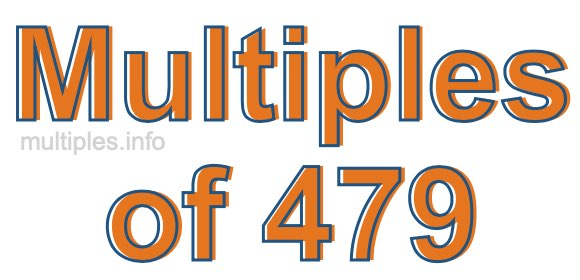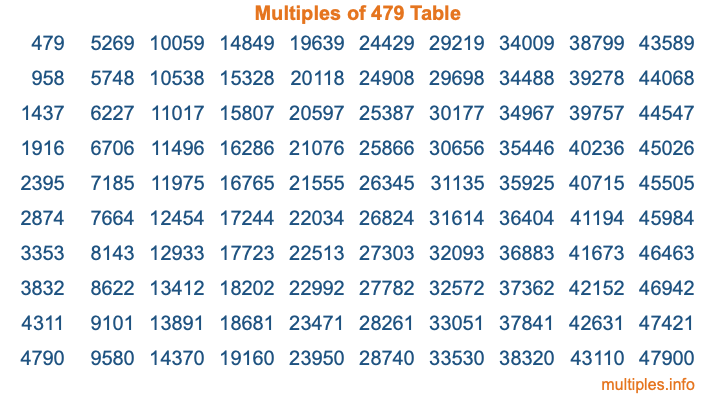Multiples of 479Welcome to the Multiples of 479 page. Here we will first teach you everything you will ever need to know about the multiples of 479, and then give you a study guide summary of everything we taught you to make sure you remember it all. Use this page to look up facts and learn information about the multiples of 479. This page will make you a multiples of four hundred seventy-nine expert!

Definition of Multiples of 479
Multiples of 479 are all the numbers that when divided by 479 equal an integer. Each of the multiples of 479 are called a multiple. A multiple of 479 is created by multiplying 479 by an integer.

Therefore, to create a list of multiples of 479, you start with 1 multiplied by 479, then 2 multiplied by 479, then 3 multiplied by 479, and so on for as long as you want. Thus, the list of the first five multiples of 479 is 479, 958, 1437, 1916, and 2395. To see a larger list of multiples of 479, see the printable image of Multiples of 479 further down on this page. We also have a category where you can choose any nth multiple of 479.

Multiples of 479 Checker
The Multiples of 479 Checker below checks to see if any number of your choice is a multiple of 479. In other words, it checks to see if there is any number (integer) that when multiplied by 479 will equal your number. To do that, we divide your number by 479. If the the quotient is an integer, then your number is a multiple of 479.

Is  a multiple of 479?

Least Common Multiple of 479 and ...
A Least Common Multiple (LCM) is the lowest multiple that two or more numbers have in common. This is also called the smallest common multiple or lowest common multiple and is useful to know when you are adding our subtracting fractions. Enter one or more numbers below (479 is already entered) to find the LCM.

Check out our LCM Calculator if you need more details about the Least Common Multiple or if you need the LCM for different numbers for adding and subtraction fractions.

nth Multiple of 479
As we stated above, 479 is the first multiple of 479, 958 is the second multiple of 479, 1437 is the third multiple of 479, and so on. Enter a number below to find the nth multiple of 479.

th multiple of 479

Multiples of 479 vs Factors of 479
479 is a multiple of 479 and a factor of 479, but that is where the similarities end. All postive multiples of 479 are 479 or greater than 479. All positive factors of 479 are 479 or less than 479.

Below is the beginning list of multiples of 479 and the factors of 479 so you can compare:

Multiples of 479: 479, 958, 1437, 1916, 2395, etc.

Factors of 479: 1, 479

As you can see, the multiples of 479 are all the numbers that you can divide by 479 to get a whole number. The factors of 479, on the other hand, are all the whole numbers that you can multiply by another whole number to get 479.

It's also interesting to note that if a number (x) is a factor of 479, then 479 will also be a multiple of that number (x).

Multiples of 479 vs Divisors of 479
The divisors of 479 are all the integers that 479 can be divided by evenly. Below is a list of the divisors of 479.

Divisors of 479: 1, 479

The interesting thing to note here is that if you take any multiple of 479 and divide it by a divisor of 479, you will see that the quotient is an integer.

Multiples of 479 Table
Below is an image of the first 100 multiples of 479 in a table. The table is in chronological order, column by column. The first column has the first ten multiples of 479, the second column has the next ten multiples of 479, and so on.The Multiples of 479 Table is also referred to as the 479 Times Table or Times Table of 479. You are welcome to print out our table for your studies.

Negative Multiples of 479
Although not often discussed or needed in math, it is worth mentioning that you can make a list of negative multiples of 479 by multiplying 479 by -1, then by -2, then by -3, and so on, to get the following list of negative multiples of 479:

-479, -958, -1437, -1916, -2395, etc.

Multiples of 479 Summary
Below is a summary of important Multiples of 479 facts that we have discussed on this page. To retain the knowledge on this page, we recommend that you read through the summary and explain to yourself or a study partner why they hold true.

There are an infinite number of multiples of 479.

A multiple of 479 divided by 479 will equal a whole number.

479 divided by a factor of 479 equals a divisor of 479.

The nth multiple of 479 is n times 479.

The largest factor of 479 is equal to the first positive multiple of 479.

479 is a multiple of every factor of 479.

479 is a multiple of 479.

A multiple of 479 divided by a divisor of 479 equals an integer.

479 divided by a divisor of 479 equals a factor of 479.

Any integer times 479 will equal a multiple of 479.

Multiples of a Number
Here you can get the multiples of another number, all with the same attention to detail as we did for multiples of 479 on this page.

Multiples of
Multiples of 480
Did you find our page about multiples of four hundred seventy-nine educational? Do you want more knowledge? Check out the multiples of the next number on our list!